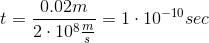# MCAT Physical : Reflection and Refraction

## Example Questions

### Example Question #1 : Reflection And Refraction

A light ray traveling through a medium is reflected by a second medium at an angle of 20⁰ to the interface between the two media.  Which of the following is true?

The refractive indices of the media must be equal

The frequency of the light wave is greater upon reflection

The angle of reflection is 70o

The refractive index of the second medium is equal to sin(20⁰)

The angle of reflection is 70o

Explanation:

The angle of reflection is the angle between the reflected light ray and a line perpendicular to the interface between the two media. The angle of reflection must be complementary to 20o.

90o – 20o = 70⁰

### Example Question #1 : Reflection And Refraction

An incandescent light bulb is shown through a glass prism. The certain wavlength of the light is then directed into a glass cuvette containing an unknown concentration of protein. Commonly, this process is called spectroscopy and is used to determine the concentrations of DNA, RNA, and proteins in solutions. The indices of reflection of air, glass, and the solution are 1, 1.5, and 1.3, respectively.

The process of light entering glass from the air is called __________.

reflection

diffraction

absorption

refraction

refraction

Explanation:

This question is asking us about the different processes that can happen to light upon hitting a surface. Thinking back to the properties of light, we know that absorption, reflection, and refraction are all processes that light can undergo when interacting with a surface.

Absorption means that the energy associated with the light is captured, and no photons are ejected from the surface after the collision of the incident photon and the surface. Reflection occurs when no light enters the new medium and instead bounces off at the angle to normal that it hit. Refraction occurs when some light enters the new medium. In this case, light is entering glass from the air; thus, the process we are concerned about is refraction.

### Example Question #3 : Reflection And Refraction

Sound projected from an opera stage strikes a flat wall in the opera house at an angle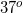to the normal. What conclusion can be drawn about the reflection of this sound from the wall back into the room?

The reflection will be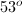to the opposite side of the normal

The reflection will beto the same side of the normal

The reflection will be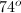to the opposite side of the normal

The reflection will be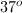to the opposite side of the normal

The reflection will beto the same side of the normal

The reflection will beto the opposite side of the normal

Explanation:

For all waves, the angle of incidence is equal to the angle of reflection. The projection is to the opposite side of the normal at the same angle as the incident wave.

Think of the wall as a mirror. The angle with which the wave impacts a mirror will be equal to the angle with which it is reflected, but mirrored across the normal.

### Example Question #12 : Optics

Glass has an index of refraction of roughly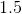. How much time would it take for light to pass through this glass if it were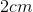thick?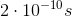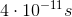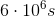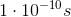Explanation:

Since the index of refraction is 1.5, we can determine the speed of light in the glass using the following equation: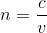Rearranged to solve for velocity: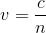Once the speed of light in the glass is known, we can use this quantity to determine how long it will take for the light to travel the width of the glass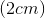.